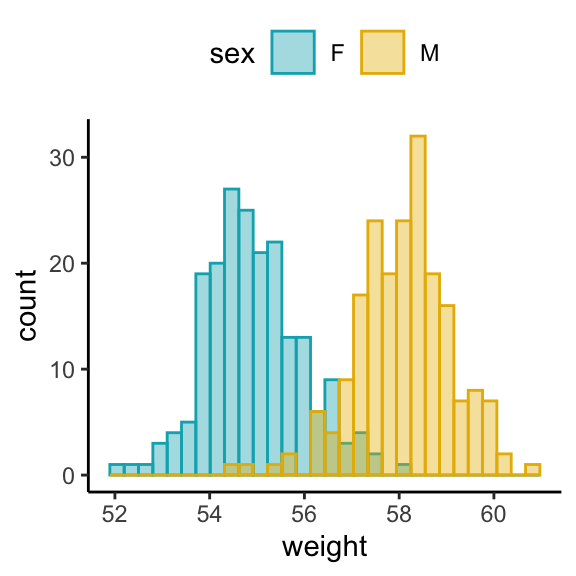# How to Create Histogram by Group in R#### How to Create Histogram by Group in R

In this article, you will learn how to easily create a histogram by group in R using the ggplot2 package.

#### Related Book

GGPlot2 Essentials for Great Data Visualization in R

## Prerequisites

• Load the ggplot2 package and set the theme function theme_classic() as the default theme:
library(ggplot2)
theme_set(
theme_classic() +
theme(legend.position = "top")
)
• Create a demo dataset: Weight data by sex:
set.seed(1234)
wdata = data.frame(
sex = factor(rep(c("F", "M"), each=200)),
weight = c(rnorm(200, 55), rnorm(200, 58))
)

head(wdata, 4)
##   sex weight
## 1   F   53.8
## 2   F   55.3
## 3   F   56.1
## 4   F   52.7

## Create histogram by group

# Change line color by sex
ggplot(wdata, aes(x = weight)) +
geom_histogram(aes(color = sex), fill = "white",
position = "identity", bins = 30) +
scale_color_manual(values = c("#00AFBB", "#E7B800"))

# change fill and outline color manually
ggplot(wdata, aes(x = weight)) +
geom_histogram(aes(color = sex, fill = sex),
position = "identity", bins = 30, alpha = 0.4) +
scale_color_manual(values = c("#00AFBB", "#E7B800")) +
scale_fill_manual(values = c("#00AFBB", "#E7B800"))